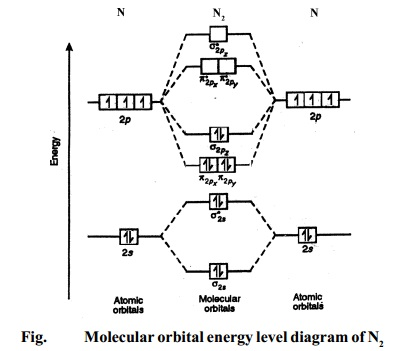Home | | Chemistry | Molecular orbital energy level diagrams -Hydrogen, Hypothetical, Nitrogen, Oxygen

# Molecular orbital energy level diagrams -Hydrogen, Hypothetical, Nitrogen, OxygenThe filling of molecular orbitals is governed by the following principles. (i)Aufbau principle (ii)Pauli's exclusion principle and (iii)Hund's rule of maximum multiplicity. Now, let us consider some examples of homo nuclear diatomic molecules.

Molecular orbital energy level diagrams of certain diatomic Homo nuclear molecules and molecular ions

The filling of molecular orbitals is governed by the following principles.

(i)Aufbau principle

(ii)Pauli's exclusion principle and

(iii)Hund's rule of maximum multiplicity. Now, let us consider some examples of homo nuclear diatomic molecules.

1. Hydrogen molecule, H2. It is formed by the combination of two hydrogen atoms. Each hydrogen atom in the ground state has one electron in 1s orbital. Therefore, in all there are two electrons in hydrogen molecule which are present in lower most s1s molecular orbital. According to Pauli's exclusion principle, these two electrons should have opposite spins.

The molecular orbital electronic configuration of hydrogen molecule is (s1s)2.

The molecular orbital energy level diagram of H2 molecule is given in Fig..The bond order of H2 molecule can be calculated as follows.

Here, Nb = 2 and Na = 0

Bond order = (Nb - Na) /2 =  2-0/2 = 2

i.                        Nature of bond : This means that the two hydrogen atoms in a molecule of  hydrogen are bonded by a single covalent bond.

ii.                        Diamagnetic character : Since no unpaired electron is present in hydrogen molecule, it is diamagnetic in nature.

2. Diatomic helium molecule, He2 (Hypothetical). The electronic configuration of helium (Z = 2) in the ground state is 1s2. As each helium atom contains two electrons, there will be four electrons in He2 molecule. Keeping in view the Aufbau principle and Pauli's exclusion principle its electronic configuration would be as follows.

He2 : (s1s)2 (s*1s)2.

The molecular orbital energy level diagram of He2 (hypothetical) is given in Fig.Here, Nb = 2 and Na = 2

Bond order = Nb - Na / 2 = 2 - 2 / 2 = 0.

As the bond order for He2 comes out to be zero, this molecule does not exist.

3. Nitrogen molecule (N2). The electronic configuration of nitrogen (Z=7) in the ground state is 1s2 2s2 2p1x 2p1y 2p1z . Therefore, the total number of electrons present in nitrogen molecule (N2) is 14. These 14 electrons can be accommodated in the various molecular orbitals in order of increasing energy.

N2 : KK(s2s )2 (s*2s )2 (P2px )2 (P2py )2 (s2pz )2

Here (s1s )2 (s*1s )2 part of the configuration is abbreviated as KK, which denotes the K shells of the two atoms. In calculating bond order, we can ignore KK, as it includes two bonding and two antibonding electrons.

The molecular orbital energy level diagram of N2 is given in Fig..The bond order of N2 can be calculated as follows.

Here,                 Nb = 8 and Na = 2

Bond order = ( Nb - Na ) / 2 =( 8 - 2)  /2 = 3.

i.                        Nature of bond : A bond order of 3 means that a triple bond is present in a molecule of nitrogen.

ii.                        Diamagnetic nature : Since all the electrons in nitrogen are paired, it is diamagnetic in nature.

4. Oxygen molecule, O2. The electronic configuration of oxygen (Z = 8) in the ground state is 1s22s22p4. Each oxygen atom has 8 electrons, hence, in O2 molecule there are 16 electrons. Therefore, the electronic configuration of O2 is as follows.

O2 : KK(s2s )2 (s*2s )2 (s2pz )2 (P2px )2 = (P2py )2 (P*2px )1 = (P*2py )1

Here (s1s )2 (s*1s )2 part of the configuration is abbreviated as KK.

The molecular orbital energy level diagram of O2 molecule is given in Fig.

Bond order = (Nb - Na ) / 2 =( 8 - 4) / 2 = 2.Study Material, Lecturing Notes, Assignment, Reference, Wiki description explanation, brief detail
11th 12th std standard Class Organic Inorganic Physical Chemistry Higher secondary school College Notes : Molecular orbital energy level diagrams -Hydrogen, Hypothetical, Nitrogen, Oxygen |